Next: Generalized Markov Decision Processes Up: Preliminaries Previous: Preliminaries

## Markov Decision Processes with the Expected Reward Criterion

The ultimate goal of decision making is to find an optimal behavior subject to some optimality criterion. Optimizing for the infinite-horizon expected discounted total reward is one of the most studied such criteria. Under this criterion, we are trying to find a policy that maximizes the expected value of, where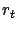is the immediate reward in time step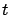and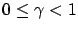is the discount factor.

A standard way to find an optimal policy is to compute the optimal value function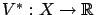, which gives the value of each state (the expected cumulated discounted reward with the given starting state). From this, the optimal policy can be easily obtained: the greedy' policy with respect to the optimal value function is an optimal policy (see e.g. [Sutton and Barto(1998)]). This is the well-known value-function approach [Bellman(1957)].

Formally, the optimal value function satisfies the following recursive system of equations known as Bellman equations [Bellman(1957)]: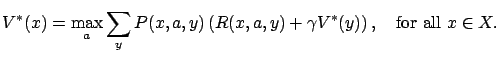(1)

Besides the state-value function, some other types of value functions can be defined as well. One example is the state-action-value function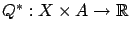, which satisfiesin the optimal case.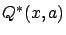has the meaning `the expected value of taking actionin state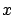while following the optimal policy''. Here the values of state-action pairs are learned instead of state values, which enables model-free learning [Watkins(1989)]. The corresponding learning algorithm is called Q-learning.

It is well known that for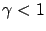, Equation 1 has a unique solution. The Bellman equations can be rewritten using operators: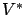is the (unique) fixed point of operator(called the dynamic programming operator), where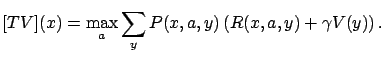Sinceis a contraction in max-norm,can be approximated by iteratively applyingto an arbitrary initial value function.Next: Generalized Markov Decision Processes Up: Preliminaries Previous: Preliminaries